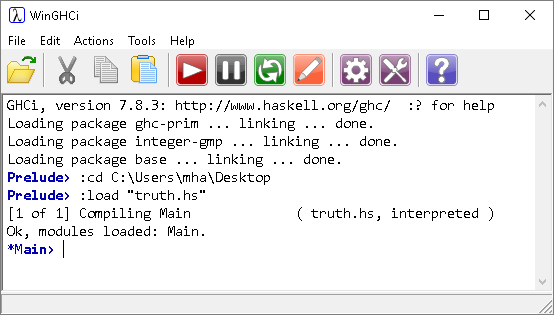# Introduction To Haskell Logical Operators

## Introduction

The Boolean values True and False are as you would expect. They are case sensitive. The operators for logic are,

• && AND
• || OR
• not NOT## Programming

We'll take this opportunity to make a little Haskell program to illustrate these operators. Type up the following code into a text editor and save with a name like truth.hs.

```main = do
putStrLn "AND FUNCTION"
putStrLn "------------"
putStrLn ""
putStrLn "A\tB\tQ"
putStrLn ("False\tFalse\t" ++ show (False && False))
putStrLn ("True\tFalse\t" ++ show (True && False))
putStrLn ("False\tTrue\t" ++ show (False && True))
putStrLn ("True\tTrue\t" ++ show (True && True))
putStrLn ""
putStrLn "OR FUNCTION"
putStrLn "------------"
putStrLn ""
putStrLn "A\tB\tQ"
putStrLn ("False\tFalse\t" ++ show (False || False))
putStrLn ("True\tFalse\t" ++ show (True || False))
putStrLn ("False\tTrue\t" ++ show (False || True))
putStrLn ("True\tTrue\t" ++ show (True || True))
```

The \t is the escape sequence for a TAB character. The show function gives us a representation of the logical operation that we can display. The brackets around the items and the use of the ++ operator will become apparent later on.

With the program saved, go to WinGHCi and load it using the File menu.

If there are no problems loading the file, the prompt will change as follows.Click on Actions, Run Main to run the program.Use brackets where you need to and you can create truth tables for all logic gates.